# 1. RoIAlign的产生背景# 3. RoI Align

• 很粗糙地选用了一个值来代替一个区域的值，而且每个区域的尺寸还有很大的差距。

$RoI Align$ 的过程如下：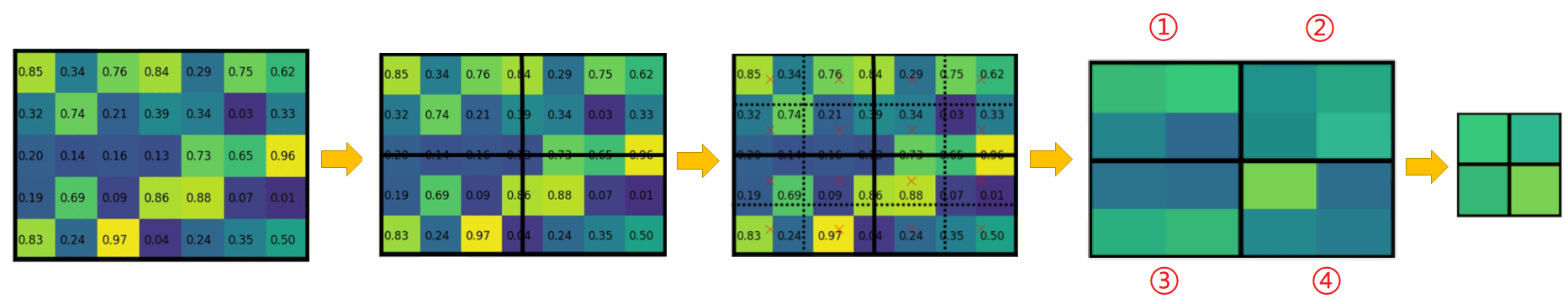• 因为最终要将这个 $5×7$ 的特征图处理成 $2×2$ 的特征图。所以先将要进行$RoI Align$ 的过程转换成 $2×2$ 个相同规模的范围，这个过程中不做任何量化处理。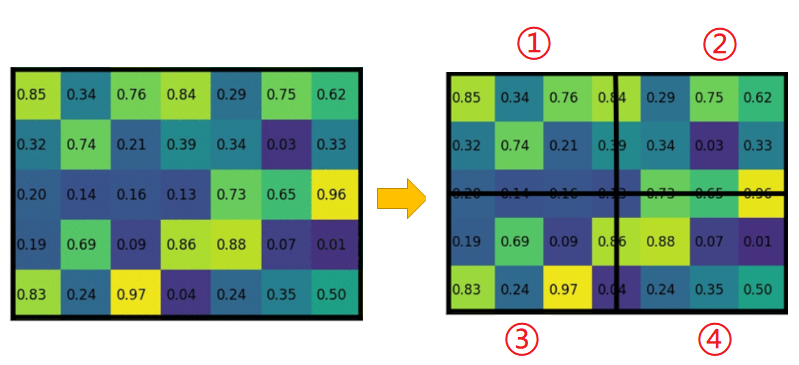• 将这 $4$ 个模块（①，②，③，④）内部同样进行这样的处理，再细分成 $4$ 个规模相同的区域（图中虚线表示）。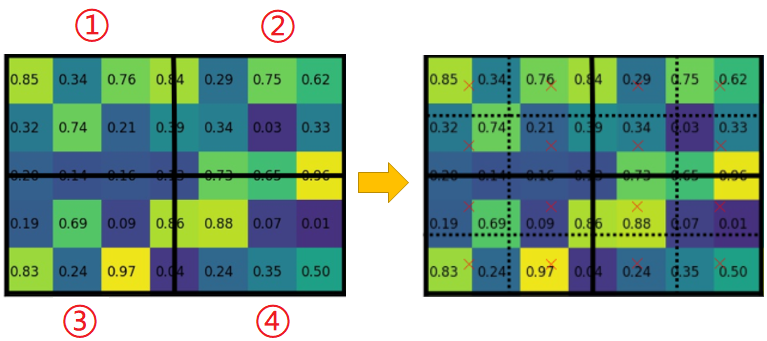• 然后对于每一个最小的区域（包含不止一个像素点）确定其中心点（图中的红色 $×$ ）然后使用双线性插值法得到这个 $×$ 号所在位置的值作为 最小格子区域 的值。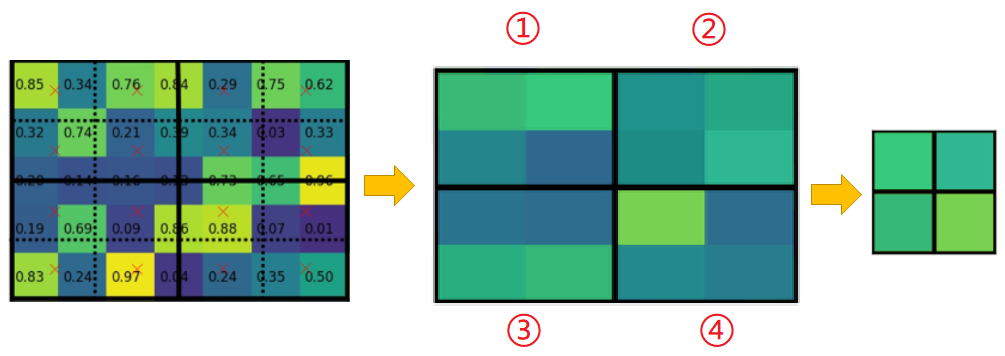• 对于每一个 小区域（①，②，③，④）都会有 $4$ 个这样的值，把这 $4$ 个值取他们的最大值作为每个 小区域（①，②，③，④） 的值。这样最终就可以得到 $4$ 个小区域的 $4$ 个值，作为最终的特征图输出结果。

$RoI Align$ 提出的这种方式可以避免过程中丢失原特征图的信息，中间过程全程不量化来保证最大的信息完整性。

# 4. 双线性插值

• 四个像素点 $Q_{11}, Q_{12},Q_{21},Q_{22}$
• 他们的坐标分别是 $(x_1,y_1),(x_1,y_2),(x_2,y_1),(x_2,y_2)$
• 他们的像素值分别是 $f(Q_{11}),f(Q_{12}),f(Q_{21}),f(Q_{22})$
• 横向插值插入的两个点 $R_1,R_2$，其坐标分别为 $(x,y_1),(x,y_2)$
• 纵向插值插入的一个点 $P$，其坐标是 $(x,y)$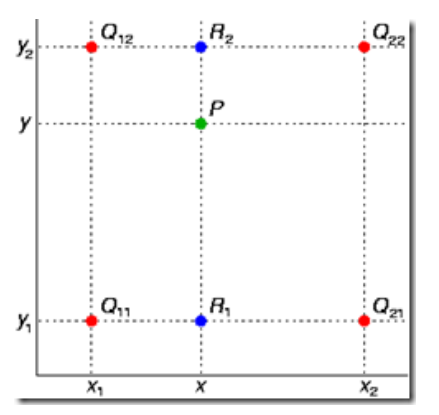插值的目的：

• 先横向插，再纵向插
• 先纵向插，再横向插

• 首先来计算横向的插值，由 $Q_{22},Q_{12}$ 得到 $R_2$ 的过程：

$\frac{f(Q_{22})-f(Q_{12})}{x_2-x_1} \approx\frac{f(Q_{22})-f(R_2)}{x_2-x}$

交叉相乘：

$({f(Q_{22})-f(Q_{12})})×(x_2-x)\approx({f(Q_{22})-f(R_{2})})×(x_2-x_1)$

化简一下：

$f(R_{2}) \approx \frac{x_2-x}{x_2-x_1}f(Q_{12})+\frac{x-x_1}{x_2-x_1}f(Q_{22})$

• 同样地，计算横向插值，由$Q_{11},Q_{21}$ 得到 $R_1$ 的过程：

$\frac{f(Q_{21})-f(Q_{11})}{x_2-x_1} \approx\frac{f(Q_{21})-f(R_1)}{x_2-x}$

计算步骤同上，化简结果：

$f(R_{1}) \approx \frac{x_2-x}{x_2-x_1}f(Q_{11})+\frac{x-x_1}{x_2-x_1}f(Q_{21})$

• 然后结合 $R_1,R_2$$y$ 方向插值得到 $P$

$\frac{f(R_{2})-f(R_{1})}{y_2-y_1} \approx\frac{f(R_{2})-f(P)}{y_2-y}$

经过化简：

$f(P) \approx \frac{y_2-y}{y_2-y_1}f(R_{1})+\frac{y-y_1}{y_2-y_1}f(R_{2})$

将得到的 $f(R_{1}),f(R_{2})$ 的化简结果带入到 $f(P)$ 的公式里面：

$f(P) \approx \frac{f(Q_{11})}{(x_2-x_1)(y_2-y_1)}(x_2-x)(y_2-y)+\frac{f(Q_{21})}{(x_2-x_1)(y_2-y_1)}(x-x_1)(y_2-y)+\frac{f(Q_{12})}{(x_2-x_1)(y_2-y_1)}(x_2-x)(y-y_1)+\frac{f(Q_{22})}{(x_2-x_1)(y_2-y_1)}(x-x_1)(y-y_1)$©️2019 CSDN 皮肤主题: 1024 设计师: 上身试试